# 1751 A Pythagorean Triple Puzzle

### Today’s Puzzle:

Even though they don’t look like it, the fourteen triangles in today’s puzzle are all Pythagorean triple triangles that most certainly have not been drawn to scale. The marked angle is the right angle for each of triangle. The hypotenuse of each triangle is less than 100. There is a chart under the puzzle listing all possible Pythagorean triples that could be used in the puzzle. No triple will be used more than once. Use logic to figure out what the missing side lengths are. Have fun!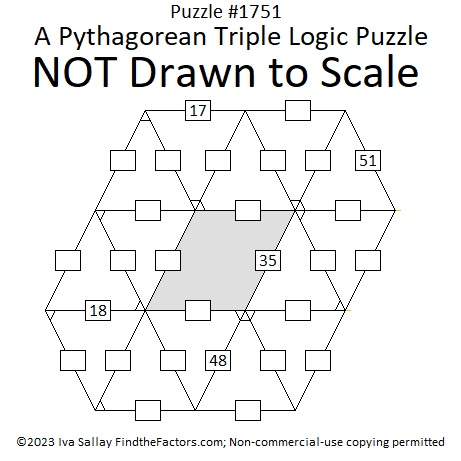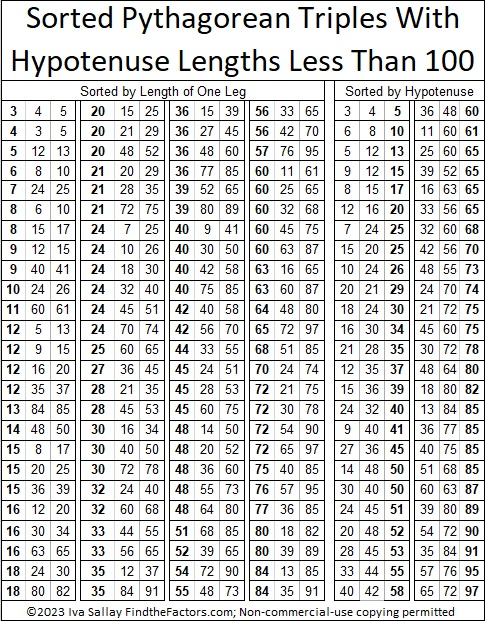### Factors of 1751:

If you remember that 51 is NOT a prime number because 3 × 17 = 51, then it isn’t difficult to recognize that 1751 also must be divisible by 17.

• 1751 is a composite number.
• Prime factorization: 1751 = 17 × 103.
• 1751 has no exponents greater than 1 in its prime factorization, so √1751 cannot be simplified.
• The exponents in the prime factorization are 1 and 1. Adding one to each exponent and multiplying we get (1 + 1)(1 + 1) = 2 × 2 = 4. Therefore 1751 has exactly 4 factors.
• The factors of 1751 are outlined with their factor pair partners in the graphic below.### More About the Number 1751:

1751 is the hypotenuse of a Pythagorean triple:
824-1545-1751 which is (8-15-17) times 103.

1751 looks interesting in some other bases:
It’s 575 in base 18 because 5(18²) + 7(18) + 5(1) = 1751,
373 base 23 because 3(23²) + 7(23) + 3(1) = 1751, and
1F1 base 35 because 1(35²) + 15(35) + 1(1) = 1751.

# 1648 A Pythagorean Triple Logic Puzzle with a Triangular Card Deck

### Today’s Puzzle:

It’s been a few years since I’ve made one of these Pythagorean triple logic puzzles. The triangles in it are shaped a little different than in years past because I also wanted to make a deck of Pythagorean triple cards that are shaped like equilateral triangles or at least as close as I can get to equal sides. This puzzle won’t be easy, but do give it a try!

PUZZLE DIRECTIONS: This puzzle is NOT drawn to scale. Although all of the angles may look like 60-degree angles, none of them are. The marked angles are 90 degrees. Lines that look parallel are NOT parallel. Although side lengths look equal, they are NOT equal. Most rules of geometry do not apply here: in fact, non-adjacent triangles in the drawing might actually overlap.

No geometry is needed to solve this puzzle. All that is needed is logic and the table of Pythagorean triples under the puzzle. The puzzle only uses triples in which each leg and each hypotenuse is less than 100 units long. The puzzle has only one solution.Print the puzzles or type the solution in this excel file: 12 Factors 1639-1648

### Triangular Card Deck of Pythagorean Triples:

You can make this deck of 50 playing cards to help you solve the puzzle or perhaps to play a domino-type game. Print each group of 25 cards on a separate sheet of paper. Cut the cards out along the solid lines and fold the cards on the dotted lines. Use a glue stick to keep the front of each card attached to its back. Laminate the cards, if desired. The right angles are the only angles marked on the cards, and the hypotenuses are all along the folded edges. Note: Some sides will not match with any other side in the deck, and the 57-76-95 triangle does not match sides with ANY triangle with a hypotenuse less than 100.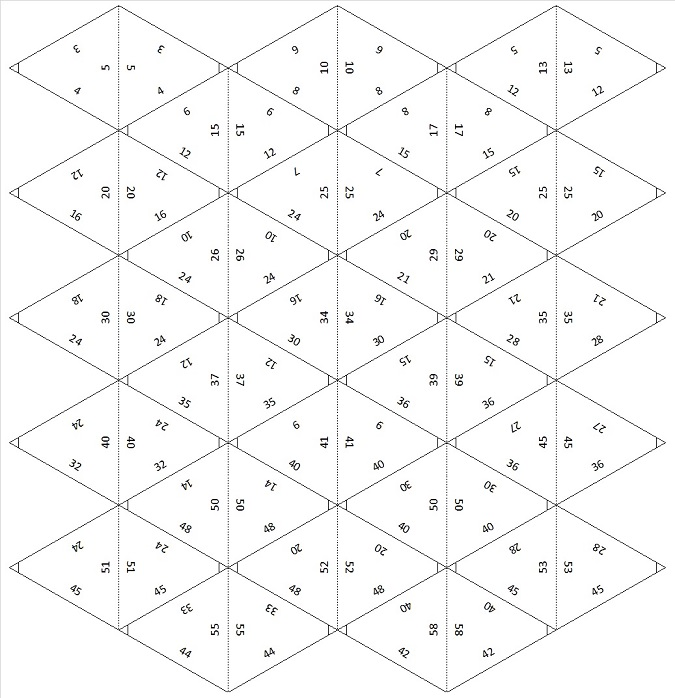### Factors of the Number 1648:

• 1648 is a composite number.
• Prime factorization: 1648 = 2 × 2 × 2 × 2 × 103, which can be written 1648 = 2⁴ × 103.
• 1648 has at least one exponent greater than 1 in its prime factorization so √1648 can be simplified. Taking the factor pair from the factor pair table below with the largest square number factor, we get √1648 = (√16)(√103) = 4√103.
• The exponents in the prime factorization are 4 and 1. Adding one to each exponent and multiplying we get (4 + 1)(1 + 1) = 5 × 2 = 10. Therefore 1648 has exactly 10 factors.
• The factors of 1648 are outlined with their factor pair partners in the graphic below.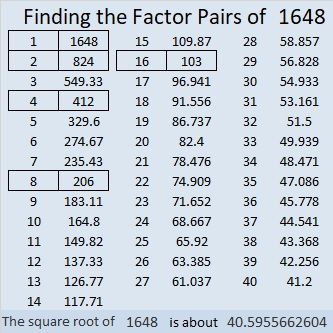### More About the Number 1648:

1648 is not the hypotenuse of any Pythagorean triples because none of its prime factors leave a remainder of 1 when divided by 4.

1648 is the difference of two squares three different ways:
413² – 411² = 1648,
208² – 204² = 1648, and
107² – 99² = 1648.

That means 1648 is a leg in THREE Pythagorean triples calculated from
413² – 411², 2(413)(411), 413² + 411²;
208² – 204², 2(208)(204), 208² + 204²; and
107² – 99², 2(107)(99), 107² + 99².

1648 can be expressed as 2(824)(1), 2(412)(2), 2(206)(4), as well as 2(103)(8).

That means 1648 is a leg in FOUR Pythagorean triples calculated from
2(824)(1), 824² – 1², 824² + 1²;
2(412)(2), 412² – 2², 412² + 2²;
2(206)(4), 206² – 4², 206² + 4²; and
2(103)(8), 103² – 8², 103² + 8².

Sometimes those formulas produce duplicate triples, but not this time. 1648 is in SEVEN Pythagorean triples!
1648-339486-339490,
1648-84864-84880,
1648-21186-21250,
1648-678975-678977,
1648-169740-169748,
1648-42420-42452,
1648-10545-10673.

Aren’t you glad that today’s puzzle was limited to triples with hypotenuses less than 100? I certainly wouldn’t want any of those triples with 1648 as a side to be part of any puzzle! But 2-digit sides, 16 and 48, helped to make today’s puzzle, and I do hope you were able to solve it today!

# 1392 and Pythagorean Triples

1392 is the hypotenuse of ONE Pythagorean triple, 960-1008-1392.

However, 1392 is the leg of so many Pythagorean triples, that it is possible I haven’t listed them all in this graphic:Why is it the hypotenuse only once, but it is a leg so many times?

Because of its factors!

• 1392 is a composite number.
• Prime factorization: 1392 = 2 × 2 × 2 × 2 × 3 × 29, which can be written 1392 = 2⁴ × 3 × 29
• 1392 has at least one exponent greater than 1 in its prime factorization so √1392 can be simplified. Taking the factor pair from the factor pair table below with the largest square number factor, we get √1392 = (√16)(√87) = 4√87
• The exponents in the prime factorization are 4, 1 and 1. Adding one to each exponent and multiplying we get (4 + 1)(1 + 1)(1 + 1) = 5 × 2 × 2 = 20. Therefore 1392 has exactly 20 factors.
• The factors of 1392 are outlined with their factor pair partners in the graphic below.1392 has only one prime factor that leaves a remainder of one when it is divided by four. That factor is 29. It makes 960-1008-1392 simply (20-21-29) times 48. Easy Peasy.

ONE of the reasons it is a leg so many times is because several of its factors are in primitive Pythagorean triples, and multiplying those triples by that factor’s factor pair gives us a triple with 1392 as a leg:

• (3-4-5) times 464 is (1392-1856-2320)
• (3-4-5) times 348 is (1044-1392-1740)
• (8-15-17) times 174 is (1392-2610-2958)
• (5-12-13) times 116
• (12-35-37) times 116, and so on

Another reason is every Pythagorean triple can be written in this form 2ab, a²-b², a²+b², and 1392 = 2(696)(1) or 2(348)(2) or 2(232)(3) or 2(174)(4) and so on.

The last reason is that since 1392 has six factor pairs in which both factors are even, it can be written as a²-b²: (The average of the two numbers in the factor pair gives us the first number to be squared. Subtract the second number from it to get the second number to be squared.)

• 696 and 2 give us 349² – 347² = 1392
• 348 and 4 give us 176² – 172² = 1392
• 232 and 6 give us 119² – 113² = 1392
• 174 and 8 give us 91² – 83² = 1392
• 116 and 12 give us 64² – 52² = 1392
• 58 and 24 give us 41² – 17² = 1392

Some of the triples can be found by more than one of the processes listed above. It can be very confusing to keep track of them all. That is why I usually only write when a number is the hypotenuse of a triple and not when it is a leg.

# Mathemagical Properties of 1105

1105 is the magic sum of a 13 × 13 magic square. Why?
Because 13×13 = 169 and 169×170÷2÷13 = 13×85 = 1105.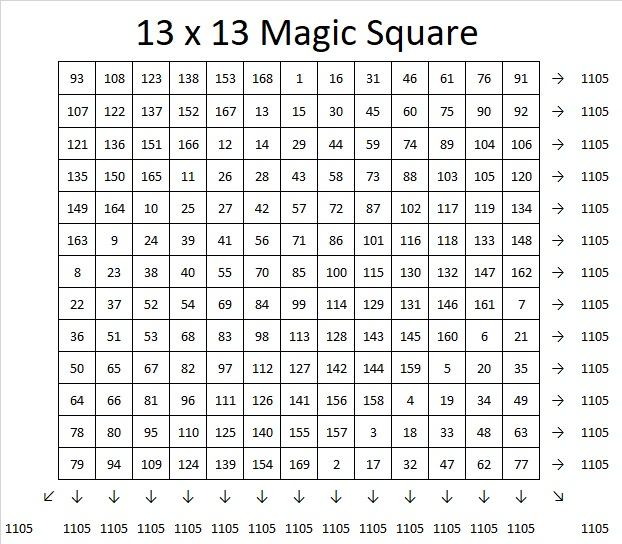If you follow the location of the numbers 1, 2, 3, 4, all the way to 169 in the magic square, you will see the pattern that I used to make that magic square. If you click on 10-factors-1102-1110  and go to the magic squares tab, you can use the same pattern or try another to create an 11 × 11, 13 × 13, or 15 × 15 magic square. The sums on the rows, columns, and diagonals will automatically populate as you write in the numbers so you can verify that you have indeed created a magic square.

1105 tiny squares can be made into a decagon so we say it is a decagonal number: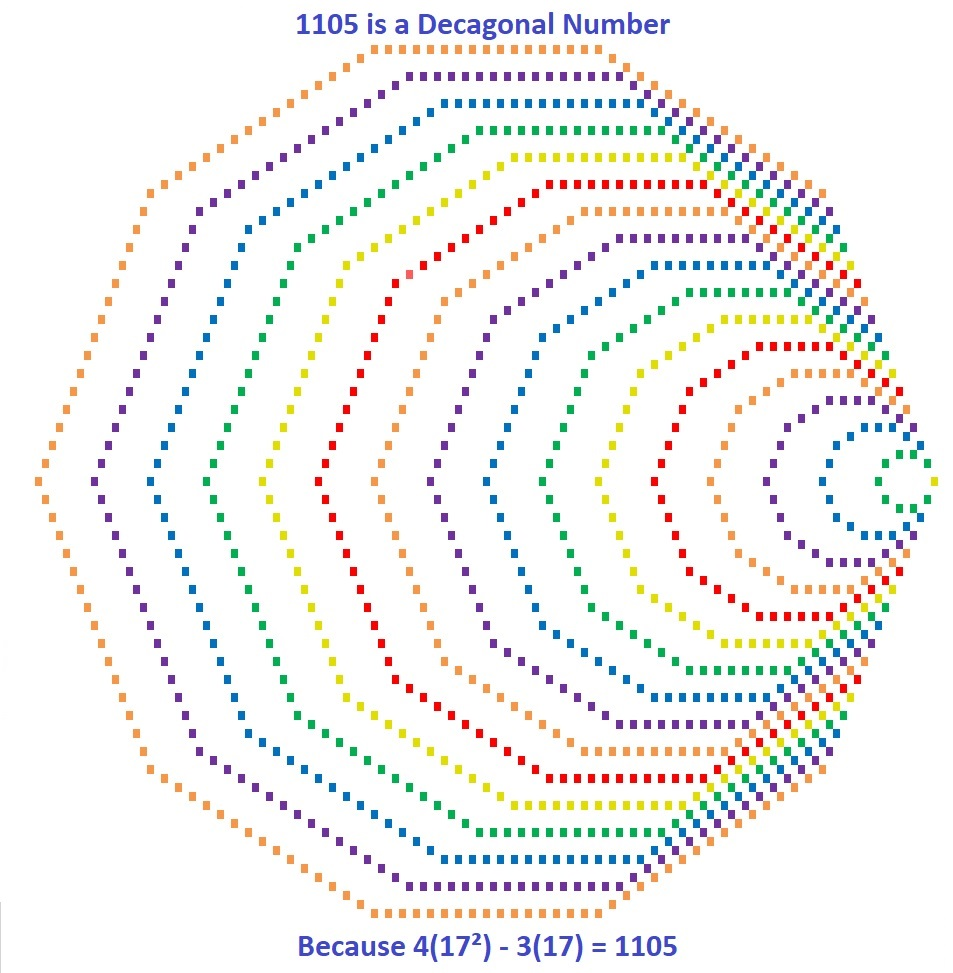Those 1105  tiny squares can also be arranged into a centered square: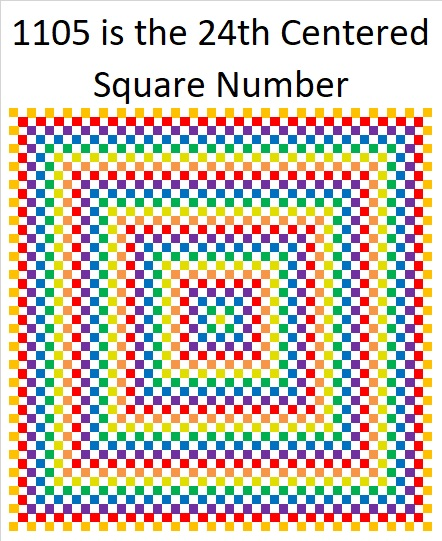Why is 1105 the 24th Centered Square Number? Because it is the sum of consecutive square numbers:
24² + 23² = 1105

But that’s not all! 1105 is the smallest number that is the sum of two squares FOUR different ways:

24² + 23² = 1105
31² + 12² = 1105
32² + 9² = 1105
33² + 4² = 1105

1105 is also the smallest number that is the hypotenuse of THIRTEEN different Pythagorean triples. Yes, THIRTEEN! (Seven was the most any previous number has had.) It is also the smallest number to have FOUR of its Pythagorean triplets be primitives (Those four are in blue type.):

47-1104-1105 calculated from 24² – 23², 2(24)(23), 24² + 23²
105-1100-1105 which is 5 times (21-220-221)
169-1092-1105 which is 13 times (13-84-85)
264-1073-1105 calculated from 2(33)(4), 33² – 4², 33² + 4²
272-1071-1105 which is 17 times (16-63-65)
425-1020-1105 which is (5-12-13) times 85
468-1001-1105 which is 13 times (36-77-85)
520-975-1105 which is (8-15-17) times 65
561-952-1105 which is 17 times (33-56-85)
576-943-1105 calculated from 2(32)(9), 32² – 9², 32² + 9²
663-884-1105 which is (3-4-5) times 221
700-855-1105 which is 5 times (140-171-221)
744-817-1105 calculated from 2(31)(12), 31² – 12², 31² + 12²

Why is it the hypotenuse more often than any previous number? Because of its factors! 1105 = 5 × 13 × 17, so it is the smallest number that is the product of THREE different Pythagorean hypotenuses.

It gets 1 triple for each of its three individual factors: 5, 13, 17, 2 triples for each of the three ways the factors can pair up with each other: 65, 85, 221, and four primitive triples for the one way they can all three be together: 1105. Thus it gets 2º×3 + 2¹×3 + 2²×1 = 3 + 6 + 4 = 13 triples.

Speaking of factors, let’s take a look at 1105’s factoring information:

• 1105 is a composite number.
• Prime factorization: 1105 = 5 × 13 × 17
• The exponents in the prime factorization are 1, 1, and 1. Adding one to each and multiplying we get (1 + 1)(1 + 1)(1 + 1) = 2 × 2 × 2 = 8. Therefore 1105 has exactly 8 factors.
• Factors of 1105: 1, 5, 13, 17, 65, 85, 221, 1105
• Factor pairs: 1105 = 1 × 1105, 5 × 221, 13 × 85, or 17 × 65
• 1105 has no square factors that allow its square root to be simplified. √1105 ≈ 33.24154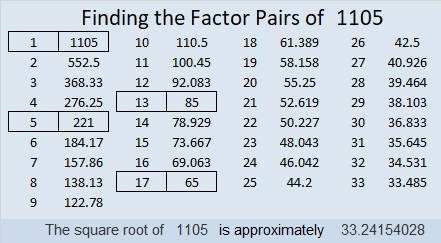1105 is also a palindrome in four different bases, and I also like the way it looks in base 8:
It’s 10001010001 in BASE 2 because 2¹º + 2⁶ + 2⁴ + 2º = 1105,
101101 in BASE 4 because 4⁵ + 4³ + 4² + 4º = 1105,
2121 in BASE 8 because 2(8³) + 1(8²) + 2(8) + 1(1) = 1105,
313 in BASE 19 because 3(19²) + 1(19) + 3(1) = 1105
1M1 in BASE 24 (M is 22 base 10) because 24² + 22(24) + 1 = 1105

Last, but certainly not least, you wouldn’t think 1105 is a prime number, but it is a pseudoprime: the second smallest Carmichael number. Only Carmichael number 561 is smaller than it is.

A Carmichael number is a composite number that behaves like a prime number by giving a false positive to all of certain quick prime number tests:
1105 passes the test p¹¹⁰⁵ Mod 1105 = p for all prime numbers p < 1105. Here is an image of my computer calculator showing 1105 passing the first five tests! Only a prime number should pass all these tests.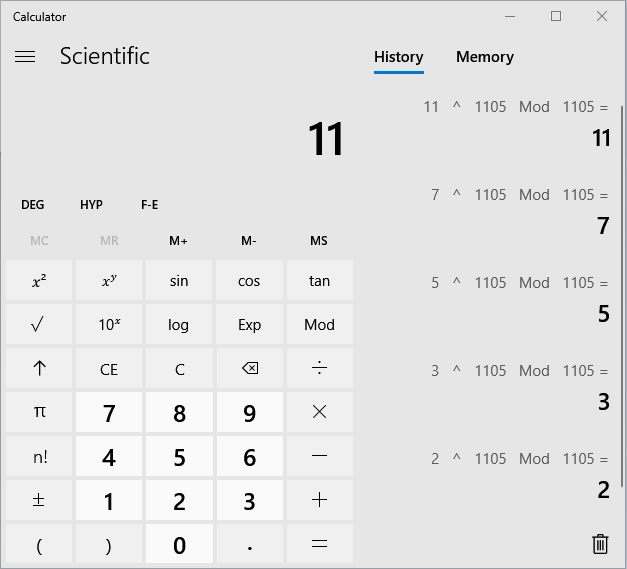1105 is indeed a number with amazing mathemagical properties!

# 865 and Level 1Print the puzzles or type the solution on this excel file: 12 factors 864-874

865 is the sum of two squares two different ways:

• 28² + 9² = 865
• 24² + 17² = 865

865 is the hypotenuse of four Pythagorean triples, two of which are primitives:

• 260-825-865, which is 5 times (52-165-173)
• 287-816-865, which is 24² – 17², 2(24)(17), 24² + 17²
• 504-703-865 which is 2(28)(9), 28² – 9², 28² – 9²
• 519-692-865, which is (3-4-5) times 173

You could see 865’s factors in two of those Pythagorean triples, and here they are again:

• 865 is a composite number.
• Prime factorization: 865 = 5 × 173
• The exponents in the prime factorization are 1 and 1. Adding one to each and multiplying we get (1 + 1)(1 + 1) = 2 × 2 = 4. Therefore 865 has exactly 4 factors.
• Factors of 865: 1, 5, 173, 865
• Factor pairs: 865 = 1 × 865 or 5 × 173
• 865 has no square factors that allow its square root to be simplified. √865 ≈ 29.41088

# How Often is 850 the Hypotenuse of a Pythagorean Triple?

We can tell if a number is the hypotenuse of a Pythagorean triple by looking at its prime factorization.

• If NONE of its prime factors leave a remainder of 1 when divided by 4, then it will NOT be the hypotenuse of Pythagorean triple.
• If at least one of its prime factors leave a remainder of 1 when divided by 4, then it WILL be the hypotenuse of Pythagorean triple.
• If ALL of its prime factors leave a remainder of 1 when divided by 4, then it will also be the hypotenuse of at least one PRIMITIVE Pythagorean triple.

850 = 2 × 5² × 17¹. Its factor, 2, prevents 850 from being the hypotenuse of a primitive Pythagorean triple, but 5² × 17¹ will actually make it the hypotenuse of SEVEN Pythagorean triples. Some of those we can find by looking at the ways we can make 850 from the sum of two squares: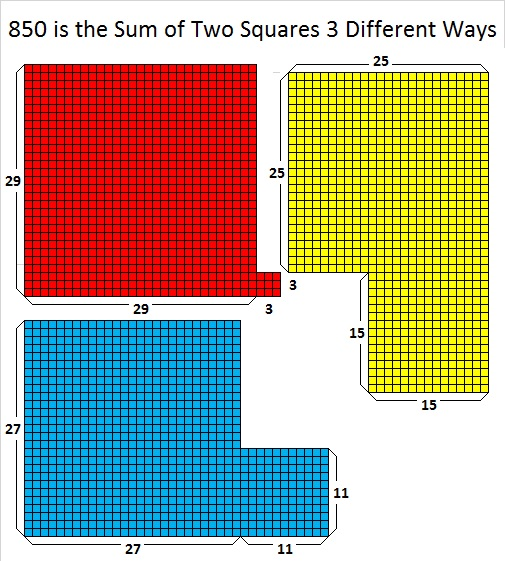29² + 3² = 850, 27² + 11² = 850, and 25² + 15² = 850

• 29² + 3² gives us 174-832-850, calculated from 2(29)(3), 29² – 3², 29² + 3², and is 2 times (87-416-425)
• 27² + 11² gives us 594-608-850, calculated from 27² – 11², 2(27)(11), 27² + 11², and is 2 times (297-304-425)
• 25² + 15² gives us 400-750-850 calculated from 2(25)(15), 25² – 15², 25² + 15², and is (8-15-17) times 50.

Let’s look a little closer at 850’s factoring information:

• 850 is a composite number.
• Prime factorization: 850 = 2 × 5 × 5 × 17, which can be written 850 = 2 × 5² × 17
• The exponents in the prime factorization are 1, 2, and 1. Adding one to each and multiplying we get (1 + 1)(2 + 1)(1 + 1) = 2 × 3 × 2 = 12. Therefore 850 has exactly 12 factors.
• Factors of 850: 1, 2, 5, 10, 17, 25, 34, 50, 85, 170, 425, 850
• Factor pairs: 850 = 1 × 850, 2 × 425, 5 × 170, 10 × 85, 17 × 50, or 25 × 34
• Taking the factor pair with the largest square number factor, we get √850 = (√25)(√34) = 5√34 ≈ 29.154759.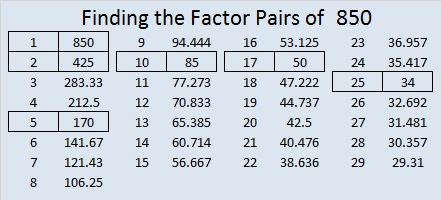Here are the SEVEN ways 850 is the hypotenuse of a Pythagorean triple with five of 850’s factor pairs in bold print:

• 174-832-850 which is 2 times (87-416-425)
• 594-608-850 which is 2 times (297-304-425)
• 510-680-850 which is (3-4-5) times 170.
• 130-840-850 which is 10 times (13-84-85).
• 360-770-850 which is 10 times (36-77-85).
• 400-750-850 which is (8-15-17) times 50
• 238-816-850 which is(7-24-25) times 34

When I wrote about 845, I said I would explore a conjecture a little more:

My conjecture: If prime numbers x and y are Pythagorean triple hypotenuses, and A and B are integers with B ≥ A and A ≥ 1, then xᴬ × y will have two primitive triples. The total number of triples xᴬ × yᴮ will have will be A + B + 2Bᴬ

So…how many Pythagorean triples does 2 × 5³ × 17¹ = 4250 have? It will have the same number as 5³ × 17¹ = 2125.

From the conjecture I figure that 2125 and 4250 will each have 1 + 3 + 2(3¹) = 10 total triples. Let’s see if I’m right …

Besides 1 and itself, the factors of 2125 are 5, 17, 25, 85, 125, and 425, all Pythagorean triple hypotenuses. Each of their respective primitive Pythagorean triples has a multiple with 4250 as the hypotenuse:

1. 850 times 5’s primitive
2. 250 times 17’s primitive
3. 175 times 25’s primitive
4. 34 times 125’s primitive
5. 50 times 85’s two primitives
6. 10 times 425’s two primitives

That’s a total of 8 Pythagorean triples from that list. We will also have triples that are 2 times 2125’s primitives. We can find those triples by looking at the sums of two squares that equal 4250.

1. 65² + 5² = 4250; but 5 is a factor of both 65 and 5, so this will produce a duplicate of one of the triples already given.
2. 61² + 23² = 4250; gives us 2806-3192-4250, calculated from 2(61)(23), 61² – 23², 61² + 23²
3. 55² + 35² = 4250; but 5 is a factor of both 55 and 35, so this will produce a duplicate of one of the triples already given.
4. 49² + 43² = 4250; gives us 552-4214-4250, calculated from 49² – 43², 2(49)(43), 49² + 43²

That gives us 2 more triples to add to the previous 8 for a total of 10 Pythagorean triples, and my conjecture still holds true.

Now one more thing about the number 850, here’s how to write it in a couple other bases:

• 505 BASE 13, because 5(13²) + 5(1) = 5(170) = 850.
• PP BASE 33 (P is 25 base 10) because 25(33) + 25(1) = 25(34) = 850

# 824 and Level 3

824 is the sum of all the prime numbers from 61 all the way to 103, which just happens to be one of its prime factors!Print the puzzles or type the solution on this excel file: 10-factors-822-828

824 is a leg in a few Pythagorean triples:

• 618-824-1030 because that is 206 times (3-4-5)
• 824-1545-1751 because that is 103 times (8-15-17)
• 824-10593-10625 because 2(103)(4) = 824
• 824-21210-21226 because 105² – 101² = 824
• 824-42432-42440 because 2(206)(2) = 824
• 824-84870-84874 because 207² – 205² = 824
• Primitive 824-169743-169745 because 2(412)(1) = 824

Five of those triples were derived directly from 824’s factor pairs.

Two of the triples were derived indirectly:

• What is (105+101)/2, (105-101)/2?
• Also, what is (207+205)/2, (207+205)/2?

The answer to both questions is a factor pair of 824.

You can read more about finding Pythagorean triples for numbers that are divisible by 4 here.

• 824 is a composite number.
• Prime factorization: 824 = 2 × 2 × 2 × 103, which can be written 824 = 2³ × 103
• The exponents in the prime factorization are 3 and 1. Adding one to each and multiplying we get (3 + 1)(1 + 1) = 4 × 2 = 8. Therefore 824 has exactly 8 factors.
• Factors of 824: 1, 2, 4, 8, 103, 206, 412, 824
• Factor pairs: 824 = 1 × 824, 2 × 412, 4 × 206, or 8 × 103
• Taking the factor pair with the largest square number factor, we get √824 = (√4)(√206) = 2√206 ≈ 28.7054# 785 and Level 4

• 785 is a composite number.
• Prime factorization: 785 = 5 x 157
• The exponents in the prime factorization are 1 and 1. Adding one to each and multiplying we get (1 + 1)(1 + 1) = 2 x 2 = 4. Therefore 785 has exactly 4 factors.
• Factors of 785: 1, 5, 157, 785
• Factor pairs: 785 = 1 x 785 or 5 x 157
• 785 has no square factors that allow its square root to be simplified. √785 ≈ 28.01785.Now for today’s puzzle:Print the puzzles or type the solution on this excel file: 12-factors-782-787

—————————————–

Here’s a little more about the number 785:

785 is the sum of two squares two different ways:

• 28² + 1² = 785
• 23² + 16² = 785

785 is also the sum of three squares three different ways:

• 26² + 10² + 3² = 785
• 25² + 12² + 4² = 785
• 19² + 18² + 10² = 785

Because its prime factorization is 5 × 157 (two numbers that are also the sum of two squares), 785 is the hypotenuse of four Pythagorean triples, two of which are primitive triples:

• 56-783-785 primitive calculated from 2(28)(1), 28² – 1², 28² + 1²
• 273-736-785 primitive calculated from 23² – 16², 2(23)(16), 23² + 16²
• 425-660-785 which is 5 times 85-132-157
• 471-628-785 which is 157 times 3-4-5

785 is also a palindrome in two different bases:

• 555 BASE 12; note that 5(144) + 5(12) + 5(1) = 785
• 101 BASE 28; note that 1(28²) + 0(28) + 1(1) = 785

—————————————–# Can You See How 779’s Factor Pairs Are Hiding in Some Pythagorean Triples?

• 779 is a composite number.
• Prime factorization: 779 = 19 x 41
• The exponents in the prime factorization are 1 and 1. Adding one to each and multiplying we get (1 + 1)(1 + 1) = 2 x 2 = 4. Therefore 779 has exactly 4 factors.
• Factors of 779: 1, 19, 41, 779
• Factor pairs: 779 = 1 x 779 or 19 x 41
• 779 has no square factors that allow its square root to be simplified. √779 ≈ 27.91057.Those factor pairs are hiding in some Pythagorean triples. Scroll down to read how, but first here’s today’s puzzle: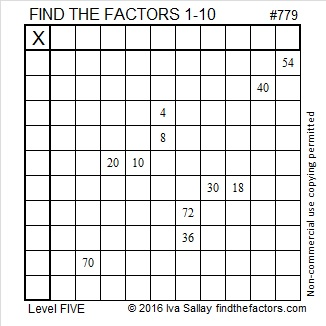Print the puzzles or type the solution on this excel file: 10-factors-2016

————————————–

And now some Pythagorean triple number theory using 779 as an example:

The factors of 779 are very well hidden in five Pythagorean triples that contain the number 779. Here’s how: 779 has two factor pairs:  19 x 41 and 1 x 779. Those factor pairs show up in some way in each of the calculations for these 779 containing Pythagorean triples:

1. 171-760-779 which is 19 times each number in 9-40-41.
2. 779-303420-303421, a primitive calculated from 779(1); (779² – 1²)/2; (779² + 1²)/2.
3. 779-7380-7421 which is 41 times each number in 19-180-181.
4. 779-15960-15979 which is 19 times each number in 41-840-841.
5. 660-779-1021, a primitive calculated from (41² – 19²)/2; 19(41); (41² + 19²)/2.

Being able to find whole numbers that satisfy the equation a² + b² = c² is one reason why finding factors of a number is so worth it. ANY factor pair for numbers greater than 2 will produce at least one Pythagorean triple that satisfies a² + b² = c². The more factor pairs a number has, the more Pythagorean triples will exist that contain that number. 779 has only two factor pairs so there are a modest number of 779 containing Pythagorean triples. All of its factors are odd so it was quite easy to find all of the triples. Here’s a brief explanation on how each triple was found:

1. 799 has one prime factor that has a remainder of 1 when divided by 4. That prime factor, 41, is therefore the hypotenuse of a primitive Pythagorean triple. When the Pythagorean triple is multiplied by the other half of 41’s factor pair, 19, we get a Pythagorean triple in which 779 is the hypotenuse.
2. Every odd number greater than 1 is the short leg of a primitive Pythagorean triple. To find that primitive for a different odd number, simply substitute the desired odd number in the calculation in place of 779.
3. Because every odd number greater than 1 is the short leg of a primitive Pythagorean triple, 19(1); (19² – 1²)/2; (19² + 1²)/2 generates the primitive triple (19-180-181). Multiplying each number in that triple by the other half of 19‘s factor pair, 41, produces a triple with 779 as the short leg.
4. Because every odd number greater than 1 is the short leg of a primitive Pythagorean triple, 41(1); (41² – 1²)/2; (41² + 1²)/2 generates the primitive triple (41-840-841). Multiplying each number in that triple by the other half of 41‘s factor pair, 19, produces a triple with 779 as the short leg.
5. Since factor pair 19 and 41 have no common prime factors, the formula (41² – 19²)/2; 19(41); (41² + 19²)/2 produces another primitive triple 660-779-1021. If they did have common factors, the factor pair would still produce a triple, but it would not be a primitive one.

Here’s some other interesting facts about the number 779:

779 is the sum of eleven consecutive prime numbers:

47 + 53 + 59 + 61 + 67 + 71 + 73 + 79 + 83 + 89 + 97 = 779.

779 can also be written as the sum of three squares six different ways:

• 27² + 7² + 1² = 779
• 27² + 5² + 5² = 779
• 23² + 15² + 5² = 779
• 23² + 13² + 9² = 779
• 21² + 17² + 7² = 779
• 21² + 13² + 13² = 779

Finally, the table below shows some logical steps that could be used to solve Puzzle #779: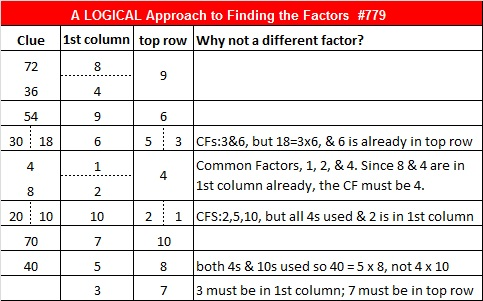# 745 Pythagorean Triple Puzzle

• 745 is a composite number.
• Prime factorization: 745 = 5 x 149
• The exponents in the prime factorization are 1 and 1. Adding one to each and multiplying we get (1 + 1)(1 + 1) = 2 x 2 = 4. Therefore 745 has exactly 4 factors.
• Factors of 745: 1, 5, 149, 745
• Factor pairs: 745 = 1 x 745 or 5 x 149
• 745 has no square factors that allow its square root to be simplified. √745 ≈ 27.294688.PUZZLE DIRECTIONS: This puzzle is NOT drawn to scale. Angles that are marked as right angles are 90 degrees, but any angle that looks like a 45 degree angle, isn’t 45 degrees. Lines that look parallel are NOT parallel. Shorter looking line segments may actually be longer than longer looking line segments. Most rules of geometry do not apply here: in fact non-adjacent triangles in the drawing might actually overlap.

No geometry is needed to solve this puzzle. All that is needed is the table of Pythagorean triples under the puzzle. The puzzle only uses triples in which each leg and each hypotenuse is less than 100 units long. The puzzle has only one solution.

Today’s Pythagorean triple puzzle has only 2 more triangles than last week’s puzzle, but it shouldn’t be any more difficult. Please, give it a try!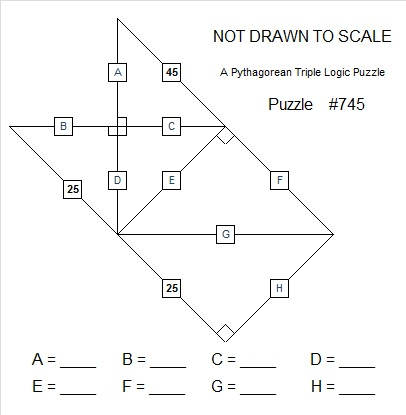Print the puzzles or type the solution on this excel file: 10 Factors 2016-01-18————————————-

Here are some fun facts about the number 745:

745 can be written as the sum of consecutive numbers three different ways:

• 372 + 373 = 745; that’s 2 consecutive numbers
• 147 + 148 + 149 + 150 + 151 = 745; that’s 5 consecutive numbers
• 70 + 71 + 72 + 73 + 74 + 75 + 76 + 77 + 78 + 79 = 745; that’s 10 consecutive numbers

745 can also be written as the sum of the thirteen prime numbers from 31 to 83. See if you can name all those primes while you add them up.

745 is the sum of two squares two different ways:

• 27² + 4² = 745
• 24² + 13² = 745

Both of 745’s prime factors are hypotenuses of Pythagorean triples, so 745 is the hypotenuse of FOUR Pythagorean triples:

• 216-713-745; calculated from 2(27)(4), 27² – 4², 27² + 4².
• 255-700-745
• 407-624-745; calculated from 2(24)(13), 24² – 13², 24² + 13².
• 447-596-745

5 is the greatest common factor of one of the non-primitive triples while 149 is the greatest common factor of the other. Which is which?

If you check any of those triples, you will see that 745² is 555025, which is a cool looking number, too.

745 is also the sum of three squares three different ways:

• 18² + 15² + 14² = 745
• 22² + 15² + 6² = 745
• 24² + 12² + 5² = 745

745 is a palindrome in three different bases, two of which are consecutive:

• 454 BASE 13; note that 4(13²) + 5(13) + 4(1) = 745.
• 3B3 BASE 14 (B= 11 base 10); note that 3(14²) + 11(14) + 3(1) = 745.
• 171 BASE 24; note that 1(24²) + 7(24) + 1(1) = 745.

————————————-